# Parallel LineSelection prerequisites: One of the following combinations of point(s) and straight object(s):

One point and one straight object

Multiple points and one straight object

One point and multiple straight objects

This Construct menu command constructs a line through each selected point parallel to each selected straight object.

 Selected Objects: Resulting Construction: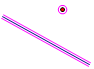One point, one straight object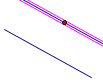One parallel line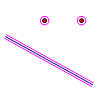Two points, one straight object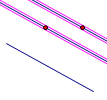Parallel lines through both points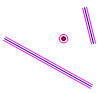One point, two straight objects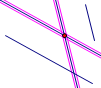Lines parallel to both straight objects